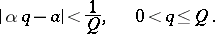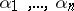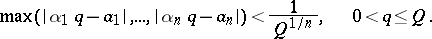# Dirichlet theorem

(diff) ← Older revision | Latest revision (diff) | Newer revision → (diff)

Dirichlet's theorem in the theory of Diophantine approximations: For any real numberand any natural numberthere exist integersandwhich satisfy the conditionWith the aid of the Dirichlet box principle a more general theorem can be demonstrated: For any real numbersand any natural numberthere exist integersandsuch that## Contents

How to Cite This Entry:
Dirichlet theorem. Encyclopedia of Mathematics. URL: http://encyclopediaofmath.org/index.php?title=Dirichlet_theorem&oldid=15058
This article was adapted from an original article by T.P. Lukashenko (originator), which appeared in Encyclopedia of Mathematics - ISBN 1402006098. See original article